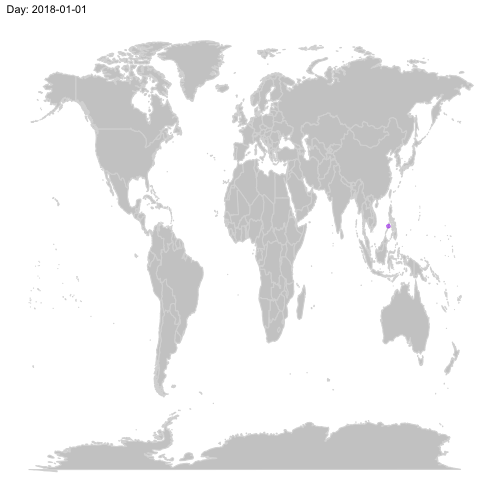# Where I was in 2018

2019-01-13

I spent a lot of time this year on the road. I started 2018 on a tropical beach in the Phillipines and ended it in Lisbon, with a lot of trips in between. Given that I spent a good part of this year playing around with maps for my thesis, I thought it would be fun to use my Google Location Services data to animate my movement this year. Here is the result:## How did I make this animation?

First, I download my Location History using Google Takeout. Then, I extracted the relevant data from the resulting JSON file using this code in R:

path <- "H.json"

full<-jsonlite::fromJSON(path)

# extracting the locations dataframe
loc <- full$locations # converting time column from posix milliseconds into a readable time scale loc$time <- as.POSIXct(as.numeric(loc$timestampMs)/1000, origin = "1970-01-01") # converting longitude and latitude from E7 to GPS coordinates loc$lat <- loc$latitudeE7 / 1e7 loc$lon <- loc\$longitudeE7 / 1e7



Subsequently, I summarised the data a little bit, filling in missing data using my calendar (my phone was broken for a month or so and didn’t record any GPS measurements).

library(ggplot2)
library(dplyr)
library(gganimate)
library(tibbletime)

locByDay <- loc  %>%
select(lat, lon, time) %>%
as_tbl_time(index = time) %>%
arrange(time) %>%
filter_time(~'2018' ) %>%  #filter to 2018
thicken(interval = "day",colname = "Day") %>%  # get each day out of the timestamp
group_by(Day) %>%
summarise(lat = mean(lat), # take the mean lattitude and longitude measurements for each day
lon = mean(lon)) %>%
pad(interval = "day") %>%  # get the missing days
mutate( # this is the missing data I am filling in manually.
lat = case_when(
(Day >=as.Date("2018-07-17") & Day <= as.Date("2018-08-16"))~47.47387, #Budapest
(Day >=as.Date("2018-08-17") & Day <= as.Date("2018-08-19"))~58.7572544, #Nykoping
(Day >=as.Date("2018-08-20") & Day <= as.Date("2018-08-21"))~59.8939529, #Oslo
(Day >=as.Date("2018-08-22") & Day <= as.Date("2018-09-15"))~47.47387, #Budapest
TRUE ~lat
),
lon = case_when(
(Day >=as.Date("2018-07-17") & Day <= as.Date("2018-08-16"))~19.04439032, #Budapest
(Day >=as.Date("2018-08-17") & Day <= as.Date("2018-08-19"))~16.9723206, #Nykoping
(Day >=as.Date("2018-08-20") & Day <= as.Date("2018-08-21"))~10.6450339, #Oslo
(Day >=as.Date("2018-08-22") & Day <= as.Date("2018-09-15"))~10.6450339, #Budapest
TRUE ~lon
)
)



Finally, I animated it using gganimate:

world <- ggplot() +
borders("world", colour = "gray85", fill = "gray80") +
theme_map()

me2018 <- world +
geom_point(aes(x = lon, y = lat),
data = locByDay,
colour = 'purple', alpha = .5
)+
transition_time(Day) +
ease_aes('linear')+
labs(title = 'Day: {frame_time}')

anim <- animate(me2018,width = 500,height = 500,nframes=365)

anim_save("me2018.gif")